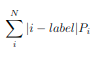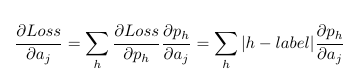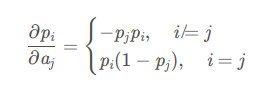# EMD gradient regarding softmax input from autograd different from theoretical value

I’m playing with simplified Wasserstein distance (also known as earth mover distance) as the loss function for N classification task. Since the gnd is a one-hot distribution, the loss is the weighted sum of the absolute value of each class id minus the gnd class id.p_i is the softmax output.
It is defined as follows:

``````class WassersteinClass(nn.Module):
def __init__(self) -> None:
super().__init__()

def forward(self, likelihood, gnd_idx):
batch_size = 1
l = likelihood.shape  # number of bins  100

gnd_idx = gnd_idx.reshape((batch_size, 1))
idxs = torch.arange(0, l, dtype=torch.float32).to(device=config.device, non_blocking=True)
batch_idxs = idxs.repeat(batch_size, 1)

loss = torch.sum(likelihood*D)
return loss
``````

Now I noticed that the gradient vanishes so I want to check its gradient regarding softmax input by creating a toy example. In this example, gnd (4) is one-hot and likelihood is nearly one-hot (peak at 2).

``````criterion = WassersteinClass()
# check gradient of softmax input
gnd_idx = torch.full(, 4)
likelihood = torch.ones(1, 5)*1e-3
likelihood[:, 2] = 100

softmax = nn.Softmax(1)
prob = softmax(likelihood)
print('sfotmax output:', prob)
>>>sfotmax output: tensor([[3.7835e-44, 1.0089e-43, 1.0000e+00, 3.7835e-44, 3.7835e-44]],

wass = criterion(prob, gnd_idx)
print('loss:', was)
``````

Its gradient regarding softmax output is quite neat.

``````b = prob.grad
>>>loss gradient after softmax: tensor([[4., 3., 2., 1., 0.]])
``````

``````a = torch.autograd.grad(wass, [likelihood])
print('loss gradient before softmax:', a) # the gradient is the distance to gnd
>>> loss gradient before softmax: (tensor([[ 7.5670e-44,  1.0089e-43,  0.0000e+00, -3.7835e-44, -7.5670e-44]]),)
``````

According to the chain rule of backpropagation``````# gradient of loss regarding softmax input a[i]
for i in range(5):